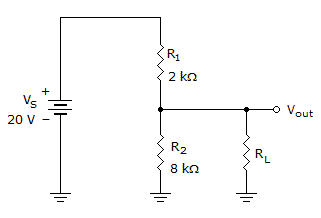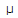# Electronics - Series-Parallel Circuits

### Exercise :: Series-Parallel Circuits - General Questions

6.

When a Wheatstone bridge is in a balanced condition, the center voltmeter in the bridge will read:

 A. twice the source voltage B. the same as the source voltage C. zero volts D. half the source voltage

Explanation:

No answer description available for this question. Let us discuss.

7.

When a load is connected to a voltage divider, the total resistance of the circuit will:

 A. decrease B. double C. increase D. remain the same

Explanation:

No answer description available for this question. Let us discuss.

8.

With 21 V applied, if R1 = 5 ohms, R2 = 35 ohms, and R3 = 14 ohms, what is the current of R2 if R1 is series connected with parallel circuit R2 and R3?

 A. 200 mA B. 800 mA C. 600 mA D. 400 mA

Explanation:

No answer description available for this question. Let us discuss.

9.

What is the total resistance of a circuit when R1 (7 k) is in series with a parallel combination of R2 (20 k), R3 (36 k), and R4 (45 k)?

 A. 4 kB. 17 kC. 41 kD. 108 kExplanation:

No answer description available for this question. Let us discuss.

10.If the load in the given circuit is 80 k, what is the bleeder current?

 A. 196A B. 1.96 mA C. 2 mA D. 2.16 mA